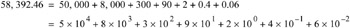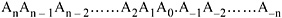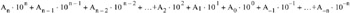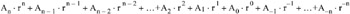Digital Circuit Design with an Introduction to CPLDs and FPGAs

# Chapter 1: Common Number Systems and Conversions

## Overview

This chapter is an introduction to the decimal, binary, octal, and hexadecimal numbers, their representation, and conversion from one base to another. The conversion procedures are illustrated with several examples.

## 1.1 Decimal, Binary, Octal, and Hexadecimal Systems

The familiar decimal number system has base or radix 10. It referred to as base 10 because it uses ten digits 0, 1, 2, 3,4, 5, 6, 7, 8, and 9. These digits are referred to as the coefficients of the decimal system. Thus, in the decimal system the coefficients are multiplied by the appropriate powers of 10 to form a number. For example, the decimal number 58, 392.46 is interpreted as:In general, any number may be represented by a series of coefficients as:In the decimal system, the A k coefficients are the ten coefficients (zero through nine), and the subscript value k denotes the power of ten by which the coefficient must be multiplied. Thus, the last expression above can also be written asDigital computers use the binary (base 2) system which has only two coefficients, 0 and 1. In the binary system each coefficient A k is multiplied by 2 k. In general, a number of base or radix r with coefficients A k is expressed asThe number 110010.01 could be interpreted as a binary, or decimal or any other base number since the coefficients 0 and 1 are valid in any number with base 2 or...